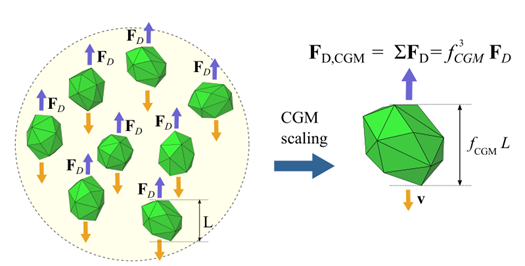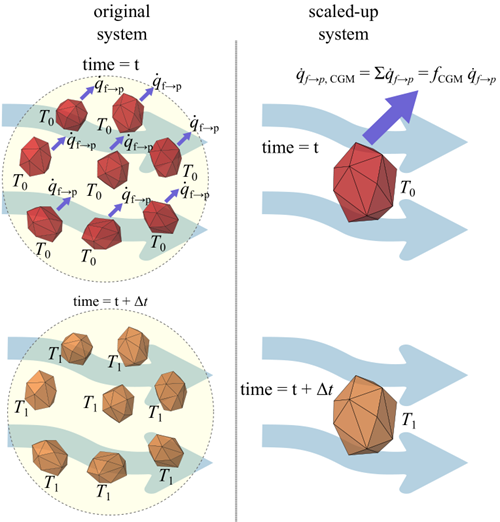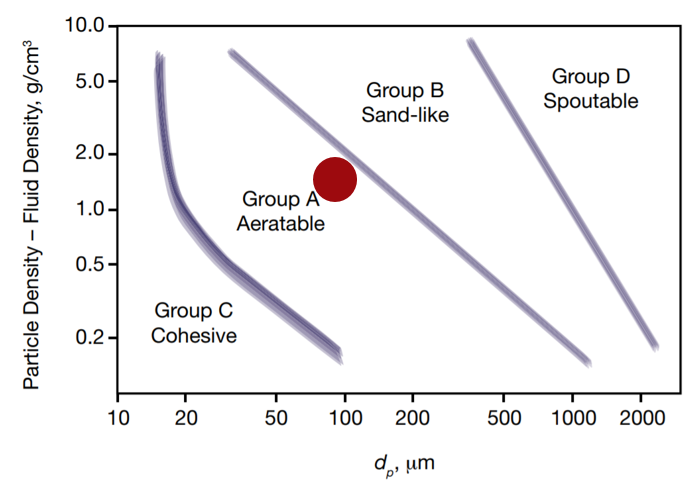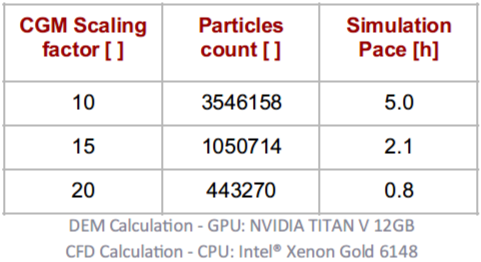## Coarse Grain Model (CGM) for CFD coupled simulations

The Coarse Grain Model (CGM) is a method used to reduce the total number of particles by using larger particles to represent groups of the original (smaller) particles while ensuring that both systems (the original and scaled-up) behave similarly.

The scaled-up particle, usually referred to as a parcel, is defined by the original particle characteristics (material, shape, size, etc.) and by a size scaling factor, fCGM. Therefore, each parcel represents fCGM3original particles.

The simulation of the coarsened particle is performed by adjusting the physical properties or DEM parameters to achieve similarity with the original particle system.

As discussed in detail in this previous post for the contact and heat transfer models, the correction, when required, is typically the inclusion of a scale-factor multiplying some of the physical properties.

## What about the fluid-particle interaction models?

When accounting for the fluid forces acting on particles as well as the convective heat transfer, adopting the CGM model for increasing the particle size is critical!

The derivation of the corrections needed for the momentum and heat exchange follows the idea that some basic characteristics of the original systems should be conserved on scaled-up systems using CGM.

For example, the terminal velocity of a group of particles falling on stagnant fluid should be the same in the original and scaled systems. By the same token, the total pressure drop in a fluid flowing through a fixed bed of particles should be the same for the original and scaled systems.

As illustrated in Figure 1, in order to achieve the same terminal velocity or similar pressure drop, the drag force acting on the coarsened particle, FD, CGM, has to be the same as the sum of the drag force FD acting on each of the original particles represented by the parcel.Figure 1. Momentum similarity constraint: in order to achieve the same terminal velocity, the drag force acting on the scaled particle (right) should be the same as the total drag force acting on the original particles (left).

The same procedure is applied to derive the corrections needed for all other non-drag fluid-particle interaction forces, such as virtual mass or lift forces.

In a similar fashion, as illustrated in Figure 2, the temperature variation of a group of hot particles falling on a stagnant cold fluid should be the same for the original and scaled systems. That can only be achieved if the heat transfer rate due to convection on the parcel is equal to the total heat transfer rate due to convection on the original particles multiplied by the number of original particles the parcel represents.Figure 2. Convective heat transfer similarity constraint: the total heat transfer rate in the coarsened particle should match the total heat transfer rate on the original particles represented by the parcel.

For more detailed information on the corrections applied to the fluid forces and convective heat transfer models when using the Coarse Grain Model approach for CFD coupled simulations, refer to the Rocky CFD Coupling Technical Manual.

## CGM limitations for coupled simulations

The particle coarsening should respect the ratio of the particle and the domain size. If the original particle size is much smaller than the characteristic size of the problem, the coarsened particle should still be considerably smaller.

For the current version, some fluid-particle interaction models are not compatible with the CGM approach. Please refer to the CFD Coupling Technical Manual for compatibility details.

## Application example

Particles from Geldart Group A are very often used in fluidized beds. However, the 1-1 simulation is challenging as a small scale model using the real particle size easily leads to billions of particles!

In this practical application example, the aim was to model a fluidized bed using particles from Geldart A group with a diameter of 0.1 mm (as mapped in the Geldart particle classification chart shown in Figure 3). If the real particle size were used in this simulation, it would lead to 3 billion particles, which is unfeasible given the computational resources available.Figure 3. Geldart particle classification chart showing that the particles used in this example belong to Group A.

The CGM model allowed the system behavior to be analyzed using a 2-way Rocky-Fluent coupled model using different scaling factors, as shown in Table 1. In this simulation, air is the fluid phase and the particles have a density of 1500 kg/m3. The Huilin & Gidaspow drag law was used to compute the drag force acting on particles.

Table 1 shows the number of particles and the simulation pace (time needed for running 1s of the simulation) for different CGM scaling factors, whereas Video 1 shows that particles behave very similarly for different scaling factors.Table 1. The number of particles and simulation pace for the fluidized bed simulation using different CGM scaling factors.

Figure 4 shows that the pressure drop between two pressure intakes matches considerably for the different scaling factors used. That is a clear indication that the drag force is correctly computed when using the CGM model. Moreover, the fluctuation behavior expected for this kind of fluidized bed is captured, and the fluctuation magnitude is similar across scaling factors.

#### References

 Bierwisch, C., Kraft, T., Riedel, H., and Moseler, M. (2009). Three-dimensional discrete element models for the granular statics and dynamics of powders in cavity filling. Journal of the Mechanics and Physics of Solids, 6257:10–31

 Cocco, R., Karri, S. R., & Knowlton, T. (2014). Introduction to fluidization. Chem. Eng. Prog, 110(11), 21-29.

### Lucilla Almeida

CAE Specialist at ESSS, D.Sc.

Lucilla holds a BE (Chemical) undergraduate degree, an M.Sc. in Chemical Engineering and a Ph.D. in Nuclear engineering from the Federal University of Rio de Janeiro. She joined ESSS in 2008 and has spent 5 years focused on applying CFD to solve common engineering problems in the Oil and Gas industry, dealing with turbulent and multiphase flow simulations. Since 2013, she is an Application Engineer for Rocky DEM Business Unit, supporting users, working on consultancy projects and validating models implemented for the CFD-DEM coupling.